# Pipe Flow Wizard Software - Pressure Drop Calculator

The Pipe Flow Wizard software calculates pressure drop in a pipe due to friction losses and fitting losses. The pressure drop in the pipe will be affected by items such as the pipe material, internal roughness of the pipe, size of diameter, length of pipe, fluid flow rate, fluid density, and fluid viscosity.

## Liquids

For liquids, the Pipe Flow Wizard software uses the Darcy Weisbach equation with accurate Colebrook-White friction factors, in order to determine the friction losses and overall pressure drop in the pipe.

## Gases

For gases, the Pipe Flow Wizard software uses Compressible Isothermal Flow equations when calculating the friction loss and total pressure drop in the pipe. The software allows the user to select which equation is used from a list that includes the General Fundamental Flow equation, Complete Isothermal Flow equation, AGA Flow equation, Weymouth Flow equation, Panhandle A Flow equation, Panhandle B Flow equation, and the IGT Flow equation.

## Pipes and Fittings

The Pipe Flow Wizard software comes with standard data (which can be added to) that allows the user to easily select pipe material, pipe diameter, pipe fittings and the fluid to be used in the calculation.Find Pressure Drop calculation and results screenshots from Pipe Flow Wizard Software for Windows.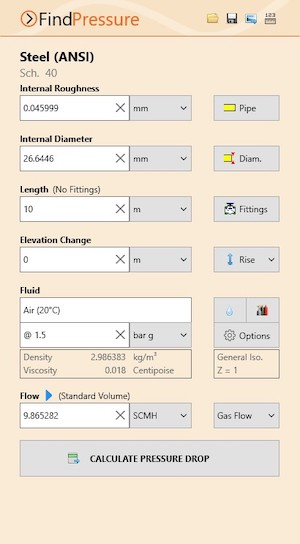Find Pressure Drop Calculation Screen (Windows)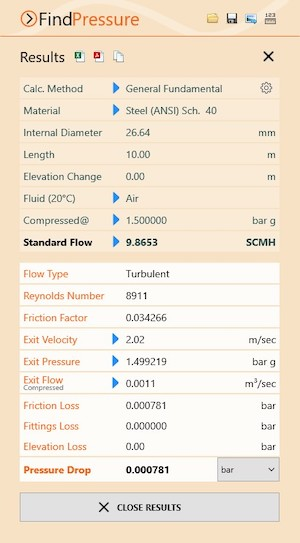Find Pressure Drop Results Screen (Windows)Find Pressure Drop calculation and results screenshots from Pipe Flow Wizard Software for macOS.Find Pressure Drop Calculation Screen (macOS)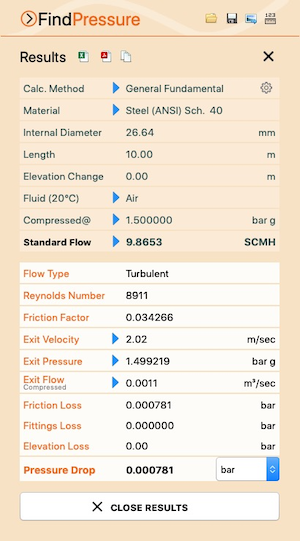Find Pressure Drop Results Screen (macOS)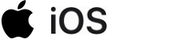Find Pressure Drop calculation and results screenshots from Pipe Flow Wizard Software for iOS.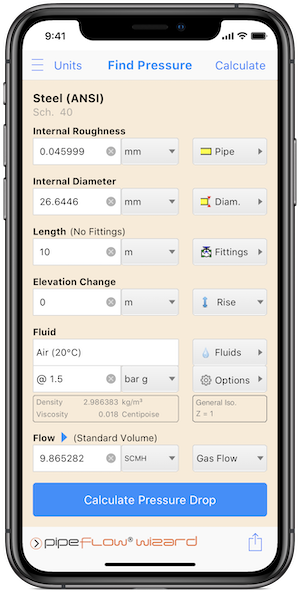Find Pressure Drop Calculation Screen (iOS)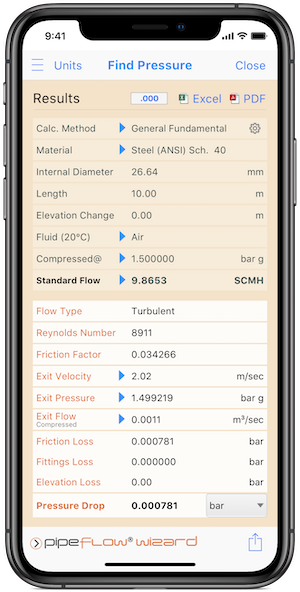Find Pressure Drop Results Screen (iOS)

Secure Online PaymentsPipe Flow Software: Piping design, Pressure drop calculator, Flow rate calculator, Pump head calculations, Pump selection software.  Copyright © Pipe Flow Software 1997-2020